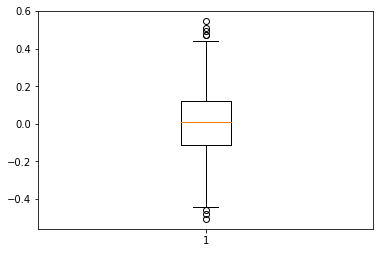In :
import pandas as pd

import numpy as np
import random

In :
a = [1,2,3,-1,-2,-3,4,-2,-2]

# version etendue

b = np.random.choice(a, size = 200, replace = True)

print(np.mean(a))
print(np.mean(b))

0.0
0.255

In :
import matplotlib.pyplot as plt
%matplotlib inline
m = []
for i in range(1000):
m.append(np.mean(np.random.choice(a, size = 200, replace = True)))

plt.boxplot(m);In :
np.mean(m)

Out:
0.0054400000000000013
In [ ]: